# CAGR

A measure of an investment’s annual growth rate over time

## What is CAGR?

CAGR stands for the Compound Annual Growth Rate. It is a measure of an investment’s annual growth rate over time, with the effect of compounding taken into account. It is often used to measure and compare the past performance of investments, or to project their expected future returns. The CAGR formula is equal to (ending value / beginning value) ^ (1/# of periods) – 1.### CAGR Formula

The Compound Annual Growth Rate formula requires only the ending value of the investment, the beginning value, and the number of compounding years to calculate. It is achieved by dividing the ending value by the beginning value and raising that figure to the inverse number of years before subtracting it by one.

The CAGR Formula is as follows: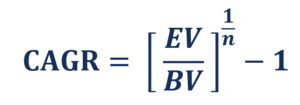Where:

EV: Ending Value

BV: Beginning Value

N: Number of Compounding Periods

### Advantages of Using the CAGR

The Compound Annual Growth rate is a useful tool for comparing a variety of investments over a similar investment horizon. One of CAGR’s advantages over an average annualized rate of return is that it is not influenced by percentage changes within the investment horizon that may yield misleading results. This advantage can be illustrated by the following example: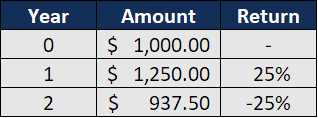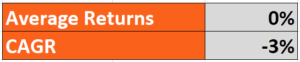The example shows that the investment gave a 25% return in the first year, raising the value from \$1,000 to \$1,250. If all capital were reinvested in the same investment vehicle for the second year, which yielded a -25% return, the value of the investment would fall to \$937.50, which is less than the initial investment amount. Although it is clearly shown that the investment generated losses over a two-year horizon, averaging returns indicates that there was no change in returns over the two years. In contrast, the Compound Annual Growth Rate shows that the investment generated negative returns over its full time horizon.

### Disadvantage of CAGR: Smoothing and Risk

One disadvantage of the Compound Annual Growth Rate is that it assumes growth to be constant throughout the investment’s time horizon. This smoothing mechanism may yield results that differ from the actual situation with a highly volatile investment.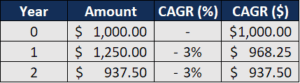Building on the above example, the Compound Annual Growth Rate correctly shows the ending value of the investment if a -3% CAGR was applied over a two-year compounding period. However, the Compound Annual Growth Rate assumes that the investment falls at a constant 3%, when, in fact, it grew by 25% in the first year.

CAGR can be used as a quick comparison tool between investment options, but any decision should be made with consideration of the trade-offs between risk and return.

### Disadvantage of CAGR: Investor Actions

Another disadvantage of the CAGR is that it does not account for the change in value caused by investor decisions to further fund or liquidate the asset. Consider the example shown in the table below: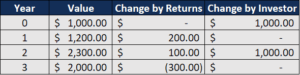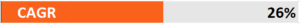As seen in this example, the source of value is important. In this case, the returns generated by the asset are zero, as the losses in the final year cancel out the gains in the first and second years. However, the value of the investment increased as a result of further injecting funds into the investment. This, in turn, raised the value of the investment although the increase in value was not generated by performance. However, the compound annual growth rate does not account for non-performance related factors in the change of value.

Check out any of the following resources to help better your understanding of investment returns and growth rates:

### Other Resources

• Terminal Growth Rate
• Investing: A Beginner’s Guide
• Financial Modeling for Beginners
• Financial Analyst Certification Program

### Financial Analyst Training

Get world-class financial training with CFI’s online certified financial analyst training program!

Gain the confidence you need to move up the ladder in a high powered corporate finance career path.

Learn financial modeling and valuation in Excel the easy way, with step-by-step training.